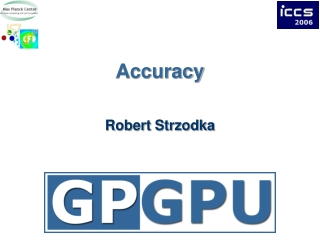Download PresentationAccuracy

# Accuracy

Télécharger la présentation## Accuracy

- - - - - - - - - - - - - - - - - - - - - - - - - - - E N D - - - - - - - - - - - - - - - - - - - - - - - - - - -
##### Presentation Transcript

1. Accuracy Robert Strzodka

2. Overview • Precision and Accuracy • Hardware Resources • Mixed Precision Iterative Refinement

3. Roundoff and Cancellation Roundoff examples for the float s23e8 format additive roundoff a= 1 + 0.00000004 =fl 1 multiplicative roundoff b= 1.0002 * 0.9998 =fl1 cancellation c=a,b (c-1) * 108 =fl 0 Cancellation promotes the small error 0.00000004 to the absolute error 4 and a relative error 1. Order of operations can be crucial: 1 + 0.00000004 – 1 =fl 0 1 – 1 + 0.00000004 =fl0.00000004

4. More Precision Evaluating (with powers as multiplications) [S.M. Rump, 1988] The correct result is -0.82739605994682136814116509547981629… for gives float s23e8 1.1726 double s52e11 1.17260394005318 long double s63e15 1.172603940053178631 This is all wrong, even the sign is wrong!! Lesson learnt: Computational Precision≠ Accuracy of Result

5. Precision and Accuracy • There is no monotonic relation between the computational precision and the accuracy of the final result. • Increasing precision can decrease accuracy ! • Even when one can prove positive effects of increased precision, it is very difficult to quantify them. • We often simply rely on the experience that increased precision helps in common cases. • But for common cases we need high precision only in very few places to obtain the desired accuracy.

6. Overview • Precision and Accuracy • Hardware Resources • Mixed Precision Iterative Refinement

7. Resources for Signed Integer Operations b: bitlength of argument, c: bitlength of result

8. Arithmetic Area Consumption on a FPGA

9. Higher Precision Emulation • Given a m x m bit unsigned integer multiplier we want to build a n x n multiplier with a n=k*m bit result • The evaluation of the first sum requires k(k+1)/2 multiplications,the evaluation of the second depends on the rounding mode • For floating point numbers additional operations for the correct handling of the exponent are necessary • A float-float emulation is less complex than an exact double emulation, but typically still requires 10 times more operations

10. Overview • Precision and Accuracy • Hardware Resources • Mixed Precision Iterative Refinement

11. Generalized Iterative Refinement

12. Direct Scheme Example: LU Solver [J. Dongarra et al., 2006]

13. Iterative Refinement: First and Second Step High precision paththrough fine nodes Low precision paththrough coarse nodes

14. Iterative Scheme Example: Stationary Solver To clarify the interaction of these two iterative schemes let us consider a general convergent iterative scheme We obtain a convergent series: [D. Göddeke et al., 2005]

15. Mixed Precision for Convergent Schemes Explicit solution representation Solution: Split the sum into a sum of partial sums (outer and inner loop). Precision reduction: Reduce the number range for G, e.g. G affine in U: Iterative refinement: this formulation is equivalent tothe refinement step in the outer iteration scheme for Problem: Summation of addends with decreasing size.

16. Iterative Convergence: First Partial Sum High precision paththrough fine nodes Low precision paththrough coarse nodes Convergent iterative scheme

17. Iterative Convergence: Second Partial Sum High precision paththrough fine nodes Low precision paththrough coarse nodes Convergent iterative scheme

18. CPU Results: LU Solver chart courtesy of Jack Dongarra

19. GPU Results: Conjugate Gradient and Multigrid

20. Conclusions • The relation between computational precision and final accuracy is not monotonic • Iterative refinement allows to reduce the precision of many operations without a loss of final accuracy • In multiplier dominated designs the resulting savings grow quadratically (area or time) • Area and time improvements benefit various architectures: FPGA, CPU, GPU, Cell, etc.